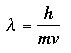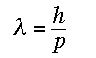# De broglie’s relationship

Start with the energy of a photon in terms of its frequency v.

E = hv

The special theory of relativity gives a new expression in terms of the velocity of light. In this expression, m refers to the relativistic mass of light which is non-zero because it is travelling with velocity c - if it were at rest, it's mass would be zero.

E = mc2

Equate these two equations and recall the expression relating the frequency and wavelength of a photon.By analogy, de Broglie argued that a particle with non-zero rest mass m and velocity v would have a wavelength given bySince mv=p, where p is the particle's momentum, the now famous de Broglie relation becomes